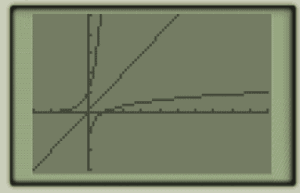# Inverse Functions: Part 1

Algebra 2 typically involves the study of inverse functions, among other topics. If you take a function and reflect it over the line y=x, you have its inverse. Let’s take y= log(x):Now, let’s add the line y=x and the inverse of y=log(x), which is y=10x. We can now see the reflection over y=x:To graph inverse functions you may simply take all the points (x,y) of the original function and swap the x coordinates for the y coordinates. For y=log(x), you can see the first two coordinates are: (1,0),(10,1). For y=10x you have (0,1) and (1,10). Thus, these are inverses.

In the next post we will learn how to find inverses algebraically.